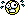A Flash Developer Resource Site

Thread: Needing Help in retrieving value from array

1. Needing Help in retrieving value from array

Hello all, any help will be appreciated
so here i got a array of number
i want to filter the array
for example
the array would be 5, 10, 20, 30 , 40 , 50 , 66 , 77
i would like to filter it it this way
if the array is between number 4 - 11 then count how many is array.. from the example above we can see there are 2 (5,10) after that store the result in textbox then continue with filtering number 12-40 and so on until the end of array..

any idea guyReply With Quote

2. Hi,

PHP Code:
var numbers:Array = new Array();
var
amounts:Array = new Array();

numbers = ;
amounts = [, , ];

for (var
i:Number 0numbers.lengthi++)
{
var
item:Number numbers[i];
for (var
j:Number 0amounts.lengthj++)
{
var
low:Number amounts[j];
var
high:Number amounts[j];
if (
item >= low && item high)
{

trace(item " is greater than " low " and lower than " high);

trace("----------");
}
}Reply With Quote

3. HI,

Expanding on that, try this, paste into a new AS2 document and test.
PHP Code:
var numbers:Array = new Array();
var
amounts:Array = new Array();
var
outcome:Array = new Array();
var

numbers = ;
amounts = [, , ];
outcome = [[], [], []];// same length as amounts length within.

for (var i:Number 0numbers.lengthi++)
{
var
item:Number numbers[i];
for (var
j:Number 0amounts.lengthj++)
{
var
low:Number amounts[j];
var
high:Number amounts[j];
if (
item >= low && item high)
{

outcome[j].push(item);

//trace(item + " is greater than " + low + " and lower than " + high);
//trace("----------");

}
}
}

for (var
q:Number 0outcome.lengthq++)
{
for (var
d:Number 0outcome[q].lengthd++)
{
if (
outcome[q].length 1)
{

addOutput += outcome[q][d] + ", ";
}
else
{

}
}

trace(outcome[q].length " are within " amounts[q] + " - " amounts[q] + " these are " addOutput);Reply With Quote

4. Hi again,

Been busy wasting time, expanding on that again.

PHP Code:
var numbers:Array = new Array();
var
amounts:Array = new Array();
var
outcome:Array = new Array();

var
i:Number 0;
var
j:Number 0;

numbers = ;
amounts = [, , , , ];

/*** fill result array with empty arrays ***/
for (0amounts.lengthi++)
{
var
populateOutcome:Array = new Array();

outcome.push(populateOutcome);
}
trace(outcome.length " arrays created inside of outcome array.");
trace("------------------------------------------");

for (
0numbers.lengthi++)
{
var
item:Number numbers[i];
for (
0amounts.lengthj++)
{
var
low:Number amounts[j];
var
high:Number amounts[j];
if (
item >= low && item <= high)
{

outcome[j].push(item);

//trace(item + " >= " + low + " && <= " + high);

}
}
}

for (
0outcome.lengthi++)
{
var
doGrammar1:String "";
var
doGrammar2:String "";

if (
outcome[i].length 2)
{

doGrammar1 " number is within number range ";

doGrammar2 " the number is ";
}
else
{

doGrammar1 " numbers are within number range ";

doGrammar2 " the numbers are ";
}

trace(outcome[i].length doGrammar1 "(" amounts[i] + " - " amounts[i] + ")\t" doGrammar2 " - [" outcome[i] + "]");Reply With Quote

5.Reply With Quote

6. I think that you guys have missed the convenient option of using array.filter(): http://board.flashkit.com/board/show...ilter()-method is an example I found discussing this.Reply With Quote

7. Hi,

Can you make an example of how it will work with this guys problem ( even though he has never come back ) nor explained any further about how he wanted the filter to continue.Reply With Quote

8. I just realized two things. 1) it would be interesting to try and make this kind of thing into a resource to make doing this kind of thing easier. 2) as2 doesn't have a built in filter. So doing it your way is really the only way. That page is still quite decent though.Reply With Quote

actionscript2, arrayPosting Permissions

• You may not post new threads
• You may not post replies
• You may not post attachments
• You may not edit your posts
•

 » Home » Movies » Tutorials » Submissions » Board » Links » Reviews » Feedback » Gallery » Fonts » The Lounge » Sound Loops » Sound FX » About FK » Sitemap